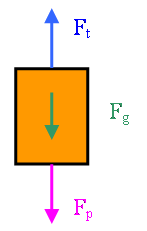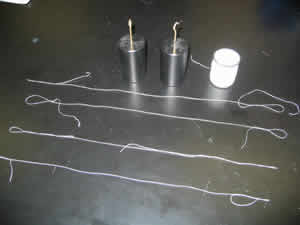## Inertia/Elasticity/Elastic Limit

Author(s): George Lyle, Gertrude Cole
Discrepant Event - Teacher's Guide
SED 695B; Fall 2005Detailed Explanation of Discrepant Event

Principles illustrated

• Newton's First Law (Inertia)
• Newton's Second Law (Proportionality of force and acceleration)
• Elasticity (Hooke's Law)
• Elastic limit

Inertia is the tendency of a massive object to remain at rest. Elasticity is the reversible strain of matter under stress. The Elastic Limit is the strain beyond which the matter is either permanently deformed or breaks. This demonstration shows all three of these effects in a dramatic fashion.

Two identical masses are suspended with two identical threads. Two additional threads are attached to the bottoms of the masses.

If the bottom thread is pulled down slowly, the combination of the weight of the mass (Fg) and the force of the downward pull (Fp) will exceed the strength of the upper thread (Ft), causing the upper thread to break. The lower thread, which does not have to support the weight of the mass, is undamaged.

The elasticity of the upper thread is evident in the slow pull demonstration, as the thread stretches considerably (in accordance with Hooke's Law) before breaking. The elastic limit is also illustrated as the upper thread fails abruptly after it stretches.

If the bottom thread is pulled down abruptly, the inertia of the mass will prevent the transfer of force to the upper thread. Newton's 2nd law comes into play as the pulling force on the thread (Fp) is attempting to accelerate the mass downward.

The force required to accelerate the mass is supplied entirely by the lower thread, and the elastic limit of the lower thread is rapidly exceeded. The small movement of the mass before the lower thread breaks is easily accommodated by the elasticity of the upper thread without exceeding its elastic limit. The small downward movement of the mass results in only a small change in the force on the upper thread (Ft) due to Hooke's Law, which states that the force is proportional to the strain.

California State Science Standards (Physics)

Motion and Forces

1. Newton's laws predict the motion of most objects. As a basis for understanding this concept:

 b. Students know that when forces are balanced, no acceleration occurs; thus an object continues to move at a constant speed or stays at rest (Newton's first law). c. Students know how to apply the law F=ma to solve one-dimensional motion problems that involve constant forces (Newton's second law).

Questioning Script

Prior knowledge & experience:
Student understands that forces can be added.

Root question:
If you pull down on the lower thread, which thread will break, the upper or the lower?

Expected Response:
The upper thread will break, because it supports both the weight of the mass and the downward pull on the lower thread.

Target response:
Which thread breaks depends upon the speed of the pull on the lower thread. A gradual pull breaks the upper thread, an abrupt pull breaks the lower.

Common Misconceptions:
Since the mass isn't moving, it cannot be accelerating, so it will transmit the entire force of an abrupt pull to the upper thread.

Prior knowledge & experience:
Student has broken thread.

Root question:
If I pull hard on the thread, will it stretch before breaking?

Expected Response:
The thread will not stretch before breaking.

Target response:
All materials have some degree of elasticity allowing them to be stretched somewhat before breaking.

Common Misconceptions:
Only "stretchy" materials like rubber are elastic.Here are the materials for the demonstration. The thread is common polyester sewing thread. The two masses are 500g and have a double hook. Not shown is a ring stand or other support from which to hang the weights. Each length of thread has a loop tied in both ends.

The thread should have a breaking strength approximately double that required to support the mass. This allows the students to clearly see the elasticity of the thread during the slower pull.A closer view of the mass and thread. Since the masses will be dropped, it is a good idea to protect the surface over which the demonstration is conducted with some sort of padding. The teacher should also consider foot protection if it is likely that the mass will fall to the floor.This is the way that the thread is tied to the mass. Note the bottom hook which makes this task a bit easier.

An online lesson on the concept of inertia.

A more complex version of this demonstration, with three possible outcomes.

A Wikipedia entry for Tensile Strength, including the concept of Elastic Limit.

Another demonstration of inertia: The Card and Marble (or coin).

Another good inertia demonstration: The Hoop and Bottle.

The classic Breaking a Cinder Block on the Teacher's Chest inertia demo.